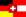Seite auf deutsch

# Mergesort

Mergesort is a very efficient sorting routine, which is patterned after the "divide and conquer"-principle and thus strongly relies on recursion.

Legend:
pink: greater value in current comparison
red: smaller value in current comparison
yellow: pivot element
orange: the part that is already sorted
blue: animation running

The idea is the following:
1. If the array contains less than two items, we are finished.
2. Otherwise we split the array into two equal-sized halves over and over and again recursively, until the subarrays under consideration have size 1;
3. Then we join two subarrays, where each subarray is sorted individually, into a large subarray, which is sorted entirely. This is the "merging" step.
The following example illustrates what happens during the merge step.
• arr = 2,3,5,4,6 consists of the individually sorted subarrays
arr1 = 2,3,5 and arr2 = 4,6.
• we allocate a temporary array of length 5 as follows:
temp = 2,3,5,6,4 - that is, arr1, followed by the reversal of arr2.
• Then we walk simultaneously from left and right through the temporary array, and write the visited values into arr, ordered by magnitude:
• 2 <= 4, thus arr  = 2; proceed the left marker by 1 from left to right. ("i++")
• 3 <= 4, thus arr  = 3; proceed the left marker by 1 from left to right. ("i++")
• 5 > 4, thus arr = 4; proceed the right marker by 1 from right to left. ("j--")
• 5 <= 6, thus arr = 5; proceed the left marker by 1 from left to right. ("i++")
• finally only 6 is left over:
6 <= 6, thus arr = 6; proceed the left marker by 1 from left to right. ("i++")
Now the indices have crossed ( j<i ), and we are finished.

The running time can be determined as follows: We have just seen that merging two individually sorted subarrays into an array of length n takes exactly n comparisons. This gives a recurrent equation for the number of comparisons V(n) needed to sort an array of length n, which roughly looks as follows:

V(n) = 2 V(n/2) + n

In order to sort an array of length n, Merge Sort recurs into two subarrays of (roughly) length n/2. After the two subarrays are sorted using V(n/2) comparisons each, we need to merge the two individually sorted subarrays into an array of length n. The merging step costs n comparisons. This recursive equation can be solved mathematically, giving a solution in O(n log n). Observe that the recurrent equation is the same for each input array. Thus the running time is O(n log n), in the best case as well as in the worst case.

#### References:

An exact analysis of the number of comparisons is found for example (in German) in:
"Steger, Angelika: Diskrete Strukturen. Band 1; Kap.4.1.1"